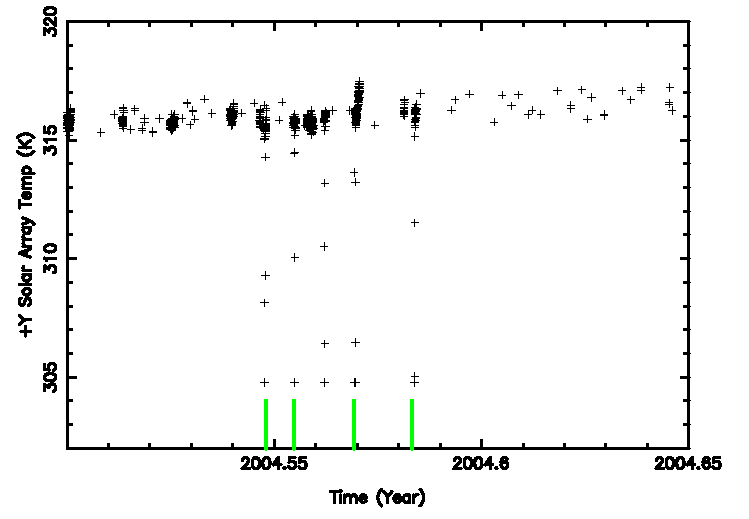## Solar Panel/Electric Power/Fine Sensor with Solar Angle

### Solar Panel Temperature and Solar Angle

To understand whether the temperatures of the solar panels change as time passes, we plotted -Y SADA temperature, +Y SADA temperature, -Y solar panel temperature, and +Y solar panel temperature against mission time. The data are divided into a 20 degree intervals of the solar pitch angle starting at 40 degrees (minimum pitch is a little greater than 46) to search for any dependencies of these quantities on the solar angle, and then each data group is plotted against the time in years.

The red lines indicate top and bottom envelopes, and an average of the data. For SADA data, all lines are estimated by a 4th degree polynomial fits to a 1 day moving box. The top envelope is the polynomial fits for the maximum values of each 1 day period, the bottom envelope is that of the minimum, and the middle line is that of the average.

The shaded area is the MTA safe operation range.

Note that the solar panel temperature drops to 304K semi-regularly every 6 months or so (some cases 3 months, and in other cases, 1 year) for a very short time (up to 2 hrs). This occurs during the Earth eclipse seasons when the tempearature is expected to go down. One example is plotted on the following plot. The green vertial lines indicate the timing of the eclipes.### Fine Sensor Temperature and Solar Angle

Fine sensor temperatures are the other quantities may behave differently with different solar angles. The plot below shows the plots for the fine sensor temperature. As before, all red lines are computed by the polynomial fits method and the shaded area is the operational range.

### Spacecraft Electric Power and Solar Angle

Similar plots are made for spacecraft bus current/voltage (ELBI/ELBV), and electric power (HRMA power/OBA power). The currents is in Amp., voltage is Volts, and the power is in Watts. Figure 3 shows the plots of these quantities of the solar angle interval between 80 and 100. As before, the red lines are the envelopes and the averages obtained by the polynomial fits.